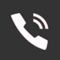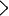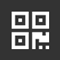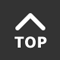## How NTC Thermistors Work

2019-09-27　 1208

1 How the semiconductor thermistor works

According to the temperature characteristics, the thermistors can be divided into two types. The temperature increase resistance increases with a positive temperature coefficient thermistor, and vice versa with a negative temperature coefficient thermistor.

(1) How does the positive temperature coefficient thermistor work?

The thermistor is made of barium titanate (BaTio3) as a basic material, and then an appropriate amount of rare earth element is added, and is sintered at a high temperature by a ceramic process. Pure barium titanate is an insulating material, but after incorporating an appropriate amount of rare earth elements such as lanthanum (La) and niobium (Nb), it becomes a semiconductor material and is called a semi-conductive barium titanate. It is a polycrystalline material with grain boundaries between the grains. For conductive electrons, the intergranular interface is equivalent to a barrier. When the temperature is low, the conductive electrons can easily cross the barrier due to the electric field in the semiconductorized barium titanate, so the resistance value is small; when the temperature rises to the Curie point temperature (ie, the critical temperature, the temperature of the element) When the control point 'General Curie Barium has a Curie point of 120 ° C), the internal electric field is destroyed and it cannot help the conductive electrons to cross the barrier, so it shows a sharp increase in the resistance value. Because this kind of component has a resistance that changes very slowly with temperature before reaching the Curie point, it has the functions of constant temperature, temperature regulation and automatic temperature control. It only generates heat, does not turn red, has no open flame, is not easy to burn, and has voltage and DC of 3 to 440V. Yes, long service life, very suitable for overheat detection of electrical equipment such as electric motors.

(2) How the negative temperature coefficient thermistor works

The negative temperature coefficient thermistor is made of a metal oxide such as manganese oxide, cobalt oxide, nickel oxide, copper oxide and aluminum oxide, and is manufactured by a ceramic process. These metal oxide materials have semiconducting properties, which are completely similar to germanium and silicon crystal materials. The number of carriers (electrons and holes) in the body is small, the resistance is high; the temperature rises, the number of carriers in the body increases, and the natural resistance The value is reduced. There are many types of negative temperature coefficient thermistors, which are used to distinguish between low temperature (-60 ~ 300 °C), medium temperature (300 ~ 600 °C), high temperature (> 600 °C), high sensitivity, good stability, fast response and long life. The advantages of low price, etc., are widely used in temperature automatic control circuits that require fixed-point temperature measurement, such as refrigerators, air conditioners, greenhouses, etc.

The combination of a thermistor and a simple amplifying circuit can detect a temperature change of one thousandth of a degree, so that a thermometer can be combined with an electronic instrument to perform high-precision temperature measurement. The general purpose thermistor operating temperature is -55 ° C ~ +315 ° C, the special low temperature thermistor operating temperature is lower than -55 ° C, up to -273 ° C.

2 The type of the thermistor

The thermistor produced in China is modeled according to the ministerial standard SJ1155-82 and consists of four parts.

The first part: the main name, the letter ‘M’ indicates the sensitive component.

The second part: category, with the letter 'Z' for the positive temperature coefficient thermistor, or the letter 'F' for the negative temperature coefficient thermistor.

Part III: Use or characteristics, expressed as a single digit (0-9). The general number '1' indicates general use, '2' indicates voltage regulation (negative temperature coefficient thermistor), '3' indicates microwave measurement (negative temperature coefficient thermistor), and '4' indicates side heating (negative temperature coefficient thermistor), '5' indicates temperature measurement, '6' indicates temperature control, '7' indicates degaussing (positive temperature coefficient thermistor), and '8' indicates linear (negative) Temperature coefficient thermistor), '9' means constant temperature type (positive temperature coefficient thermistor), '0' means special type (negative temperature coefficient thermistor)

The fourth part: serial number, also represented by numbers, represents specifications and performance.

Often, in order to distinguish the special needs of this series of products, manufacturers add a 'derived serial number' after the serial number, which is a combination of letters, numbers and ‘-’.

Example: M Z 1 1

Serial number

General purpose

Positive temperature coefficient thermistor

Sensitive component

3 main parameters of the thermistor

The operating conditions of various thermistors must be within the allowable range of their factory parameters. There are more than ten main parameters of the thermistor: nominal resistance value, ambient temperature (maximum operating temperature), measured power, rated power, nominal voltage (maximum working voltage), operating current, temperature coefficient, material constant, time Constants, etc. The nominal resistance value is the resistance value at zero power at 25 ° C. In fact, there is always a certain error, which should be within ±10%. Ordinary thermistors have a wide operating temperature range and can be selected from -55 ° C to +315 ° C as needed. It is worth noting that the maximum operating temperature of different types of thermistors varies greatly, such as MF11 sheet-like negative temperature coefficient heat. The varistor is +125 ° C, while the MF 53-1 is only +70 ° C. Students should pay attention to the experiment (generally do not exceed 50 ° C).

••0754-82484152/0754-82484153
••